## Example Questions

### Example Question #1 : How To Multiply Even Numbers

Ifis even, andis odd. Which of the following must be odd?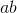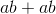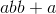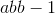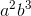Explanation:

To solve, pick numbers to representand. Let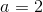and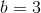. Now try each of the equations given: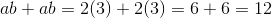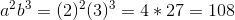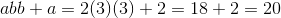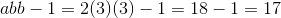Onlyworks and is thus our answer.

### Example Question #2 : How To Multiply Even Numbersis even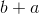is even

Quantity A:

The remainder of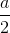Quantity B:

1

Quantity B is larger.

The relationship between the quantities cannot be determined.

The two quantities are equal.

Quantity A is larger.

Quantity B is larger.

Explanation:

Begin by considering our options.  For, it is either the case that:is even andis odd, or,is even andis even, or,is odd andis even

Now, for, it is either the case that:andare even, or,andare odd

Now, for, it cannot be the case that both are odd.  This means that the only viable option foris the case when both are even.

Therefore,must be even, meaning that the remainder ofmust be.

Therefore, quantity B is larger.

### Example Question #3 : How To Multiply Even Numbersis even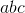is even

Therefore, which of the following must be true about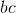?

It must be even.

It must be odd.

It could be either even or odd.

It could be either even or odd.

Explanation:

Recall that when you multiply by an even number, you get an even product.

Therefore, we know the following from the first statement:is even oris even or bothandare even.

For the second, we know this:

Sinceis even, therefore,can be either even or odd. (Regardless of what it is, we can get an even value for.)

Based on all this data, we can tell nothing necessarily about. Ifis even, thenis even, even ifis odd. However, ifis odd whileis even, thenwill be even.

### Example Question #4 : How To Multiply Even Numbers

In a group of philosophers,are followers of Durandus. Twice that number are followers of Ockham. Four times the number of followers of Ockham are followers of Aquinas. One sixth of the number of followers of Aquinas are followers of Scotus. How many total philosophers are in the group?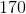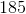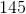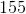Explanation:

In a group of philosophers,are followers of Durandus. Twice that number are followers of Ockham. Four times the number of followers of Ockham are followers of Aquinas. One sixth of the number of followers of Aquinas are followers of Scotus. How many total philosophers are in the group?

To start, let's calculate the total philosophers:

Ockham:* <Number following Durandus>, or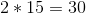Aquinas:* <Number following Ockham>, or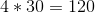Scotus:divided by, orTherefore, the total number is: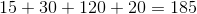### Example Question #5 : How To Multiply Even Numbers

Assumeandare both even whole numbers.

What is a possible solution for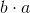?Explanation:

When two even numbers are multiplied, they must equal an even number. Also, since both variables are said to be even whole numbers, the answer must fit the requirement that its factors are two even numbers multiplied by one another. The only answer that fits both requirements iswhich can be factored into the even whole numbersand.

### Example Question #1 : How To Multiply Even Numbers

Which of the following integers has an even integer value for all positive integers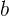and?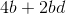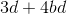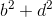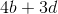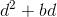There are certain patterns that can be used to predict whether the product or sum of numbers will be odd or even. The sum of two odd numbers is always even, as is the sum of two even numbers. The sum of an odd number and an even number is always odd. In multiplication the product of two odd numbers is always odd. While the product of even numbers, as well as the product of odd numbers multiplied by even numbers is always even. So for this problem we need to find scenarios where the only possibile answers are even.can only result in even numbers no matter what positive integers are used forand, because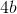must can only result in even products; the same can be said for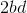. The rules provide that the sum of two even numbers is even, sois the answer.